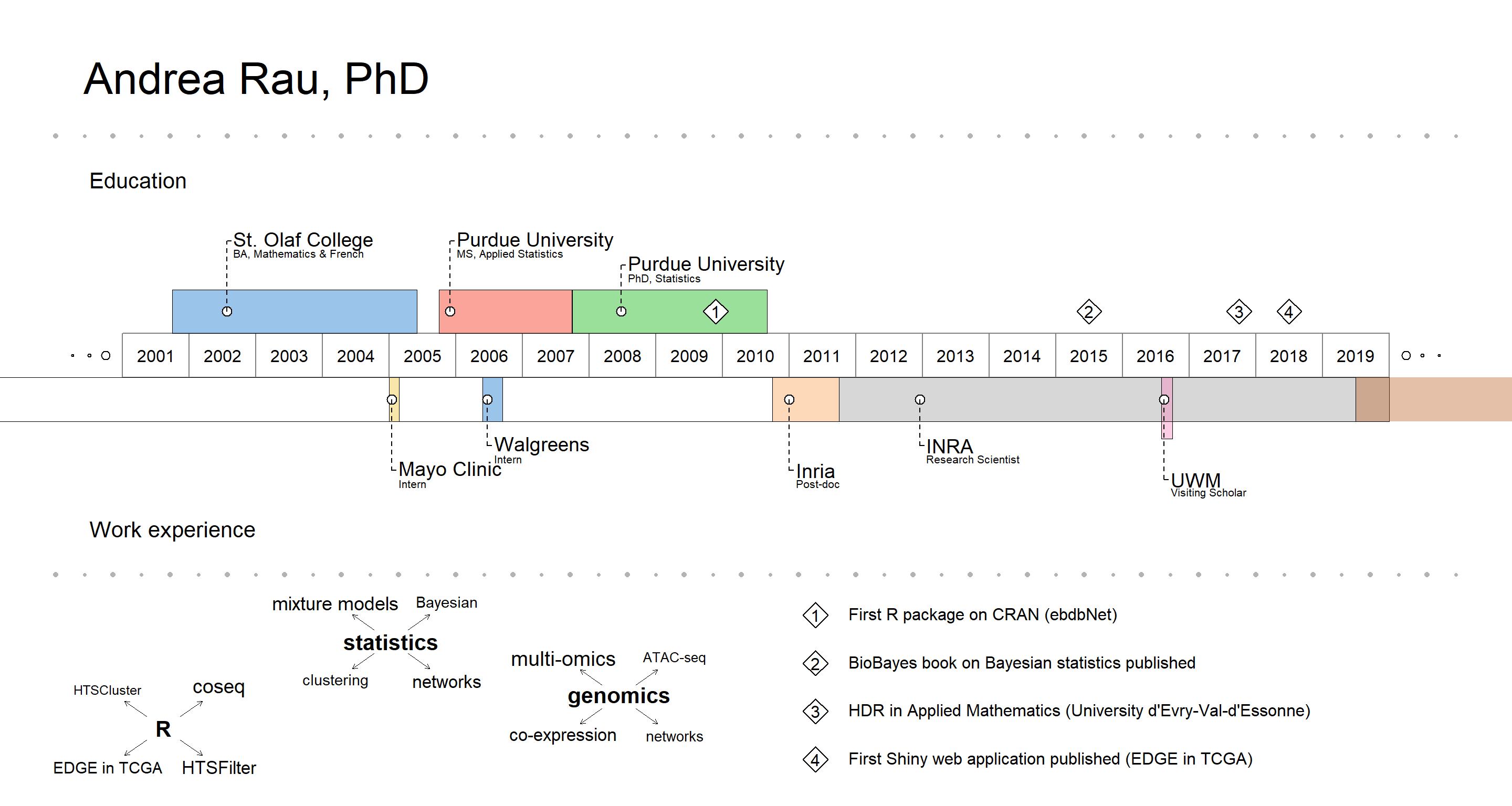This is a short post to provide details on how I created the visual CV that is included on my homepage. I got the idea for doing this from a tweet from the awesome Mara Averick about an R package called VisualResume by Nathaniel Phillips:

Once VisualResume is installed from GitHub (via devtools) and loaded, I just modified the Walter White example, resized the plot directly in the RStudio plot window, and exported to PNG.

library(VisualResume)
VisualResume::VisualResume(
titles.left = c("","Andrea Rau, PhD",  ""),
titles.right = c("", "", ""),
titles.left.cex = c(1,  4, 1),
timeline.labels = c("Education", "Work experience"),
timeline = data.frame(title = c("St. Olaf College", "Purdue University",
"Purdue University", "Inria", "INRA", "UWM", "UWM",
"Mayo Clinic", "Walgreens"),
sub = c("BA, Mathematics & French", "MS, Applied Statistics",
"PhD, Statistics", "Post-doc",
"Research Scientist", "Visiting Scholar",
"AgreenSkills+ Fellow", "Intern", "Intern"),
start = c(2001.75, 2005.75, 2007.75, 2010.75, 2011.75,
2016.58, 2018783, 2005, 2006.4),
end = c(2005.42, 2007.74, 2010.67, 2011.75, 2020, 2016.75,
2019.5, 2005.15, 2006.7),
side = c(1, 1, 1, 0, 0, 0, 0, 0, 0)),
milestones = data.frame(title = c("BA", "MS", "PhD"),
sub = c("Math/\nFrench", "Applied\nStatistics",
"Statistics"),
year = c(0, 0, 0)),
events = data.frame(year = c(2009.9, 2015.5, 2017.75, 2018.5),
title = c("First R package on CRAN (ebdbNet)",
"BioBayes book on Bayesian statistics published",
"HDR in Applied Mathematics (University d'Evry-Val-d'Essonne)",
"First Shiny web application published (EDGE in TCGA)")),
interests = list("R" = c(rep("coseq", 4), "HTSCluster",
rep("HTSFilter", 3), rep("EDGE in TCGA", 2)),
"statistics" = c(rep("mixture models", 6),
rep("clustering", 3), rep("networks", 5),
rep("Bayesian", 3)),
"genomics" = c(rep("multi-omics", 8), rep("ATAC-seq", 2),
rep("co-expression", 6), rep("networks", 3))),
year.steps = 1
)Posted on:
October 31, 2018
Length: